This video was brought to you by GeneralPAC.com, making power systems Intuitive, Open and Free for Everyone, Everywhere. Consider subscribing and supporting through patreon.com/GeneralPAC. This is a mechanism for you to support us financially so we can continue making high quality power system video tutorials. Our corporate sponsor for this topic is AllumiaX.com from Seattle, Washington. Contact them for industrial and commercial power system studies.

Introduction to Per-Unit Systems Part 1b.

Per unit conversion formulas and base values. In the previous video, Part 1a, we saw what per unit systems are and where they are used? In this video, Part 1b, we will be discussing the formulas for converting values into their per unit equivalents and we will also have a look at the term ‘Base values’.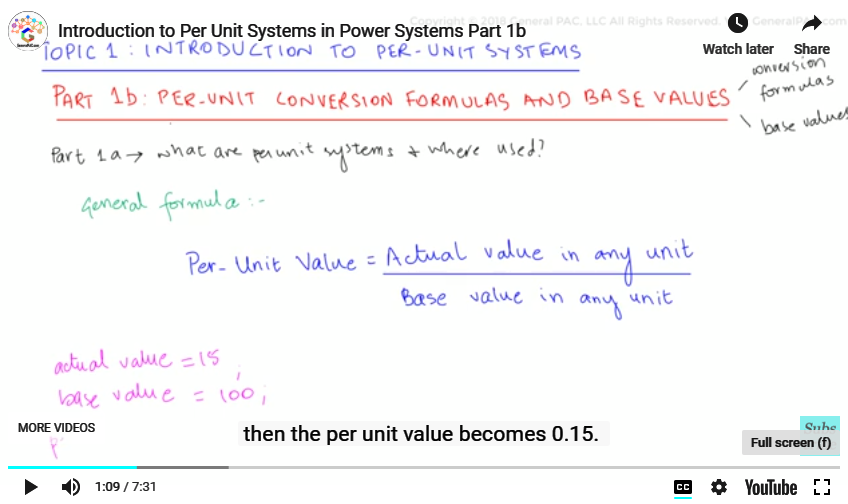The general formula that is used to calculate per unit values is:

Per Unit Value =
Actual value in any unit / Base value in any unit

For example, consider taking an actual value of 15 and a base value of 100, then the per unit value becomes 0.15. This is just a simple example and 15 and 100 are just numbers. In power engineering, these numbers can take the form of voltage, current, impedance and power. Which means that

Per Unit Value of Voltage =
Actual value of Voltage / Base value of Voltage

Similarly:

Per Unit Value of Current =
Actual value of Current / Base value of Current

And the same goes for impedance and Power.

Let’s dig deeper into these parameters. Actual values and Base values, both are in the same units which means that per unit values have no units because the original units were cancelled out with each other because of division, leaving the answer, essentially dimensionless, and so we call them per unit values p.u depicting the per unit.

Now, taking one of the formulas above, for instance, voltage. Both the numerators and denominators have units as ‘volts’ which get cancelled out, leaving us with a dimensionless per unit value. So, we can write whatever the value is like 0.1, 0.2, 0.6 etc. in p.u or per units.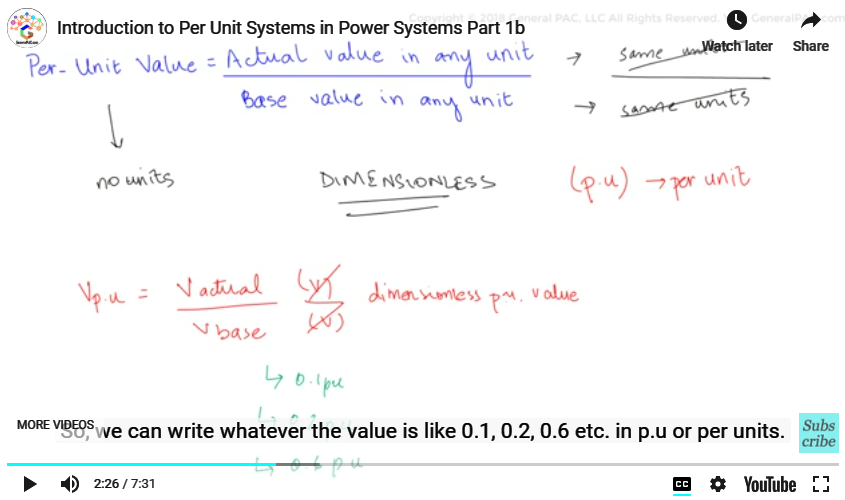Coming to the base values. Now, base values are rated values of the equipment, like ratings of a generator or a transformer. Normally, we select base values of voltage and the apparent power to carry out per unit calculations. In power systems that consists of many equipment like generators, transformers, transmission lines, capacitor banks and so forth, we take the largest equipment rating as the base value.

Now, to convert a power system into a per unit equivalent, we require four values that are the voltage base, the current base, the impedance base and the power base. However, at many instances, we may come across the ratings of generators or any other equipments as 100 V, 330 VA or 200 V or 100 A, meaning only two base values are given. But we don’t have to worry by using these given base values we can actually calculate all four base values!

Now, let’s understand this by way of an example from the book called Electric Machinery Fundamentals by Stephen Chapman (Edition no. 4).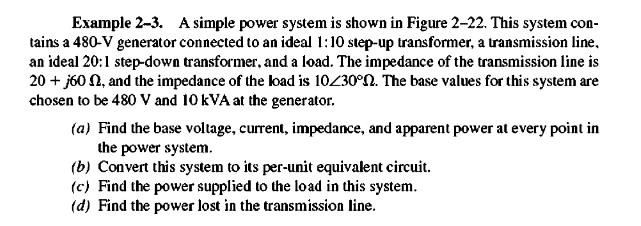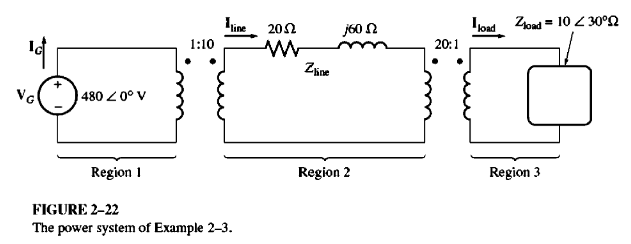Example 2.3, pg 95, Electric Machinery Fundamentals by Stephan J. Chapman (Edition 4)

Now, in this example we are given a generator in Region 1 which is at 480 volts at 0 degrees and there is a step-up transformer in Region 2 that has a transmission line with a 20 ohms resistance and 60 ohms reactance. And then there is step-down transformer which leads us to Region 3 which has a load of 10 ohms at 30 degrees. Now, in this example, we are told that the base values of the system are 480 volts and 10 KVA at the generator and in Part (a), we are asked to find the base voltage, current, impedance and the apparent power at every point in the power systems and Part (b) is to convert the system into per unit equivalents. Part (c) find the power supplied to a load in this system and Part (d) find the power lost in the transmission line.

Now, since this video is served as an introduction, we will solve only Part (a) and Part (b) for Region 1 only. The remaining parts will be solved in the next video.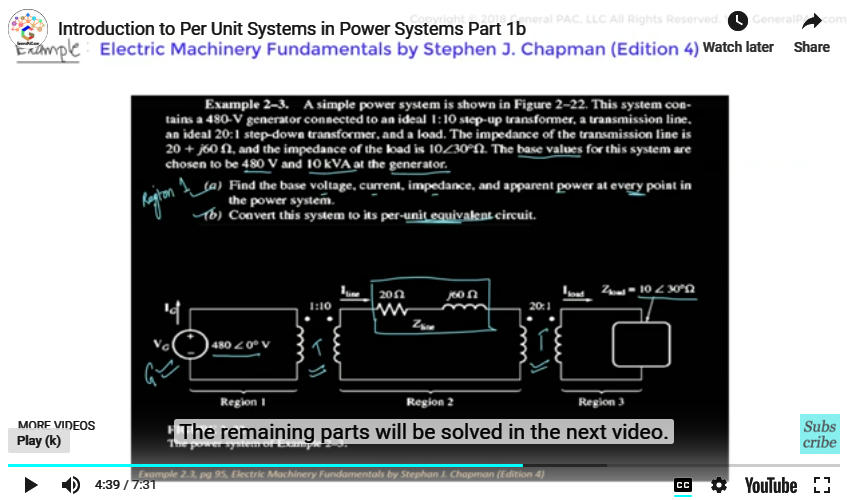Part (a) asks us to calculate the base values for current and impedances for Region 1.

As given in the question, we know that

Vbase = 480 V

Sbase = 10 kVA

We know that to calculate Ibase and Zbase, we have the following formulas:

Base current =
Base power / Base voltage

Base impedance= 〖(Base voltage)〗^2/(Base power)

Base impedance=
(Base voltage) 2 / Base power

By putting the values of Vbase and Sbase in these equations, we can calculate Ibase and Zbase.

Base current=
Base power / Base voltage
=
Sbase / Vbase
=
10 kVA / 480 V
=2 0.83 A
Base impedance =
(Base voltage) 2 / Base power
=
(Vbase) 2 / Sbase
=
(480 V) 2 / 10KVA
= 23.04 Ω

So, we have our answers as

Ibase = 20.83 A

Zbase=23.04 Ω

By solving this above part, we conclude that a minimum of two base quantities can help us find all of the four base values and then we can convert a system into its per unit equivalent system.

Moving on to part (b)

Part (b) Converting Region 1 into its per unit equivalent.

Since Region 1 consists of generating station, we would have voltage in per unit. We will use the per unit formula to find out Vpu.

Vp.u
actual voltage of voltage source in Region 1 / base voltage in Region 1

Since Region 1 is the generator side, the base voltage is 480V.

pp.u
actual voltage of voltage source in Region 1 / base voltage in Region 1
480 / 480
= 1.0 p.u

Finally, we have our answers to the easy part of the question.

.

Now, in this video we saw how to calculate base values and per unit values. And we solved an easy example to clear our concepts. In Part 1c, we will complete this example.

Now, we hope that you have a continued interest in this topic and series as a student or professional. And we also hope you find this content useful and enlightening. Please consider subscribing to GeneralPAC.com and becoming our patron at patreon.com/GeneralPAC.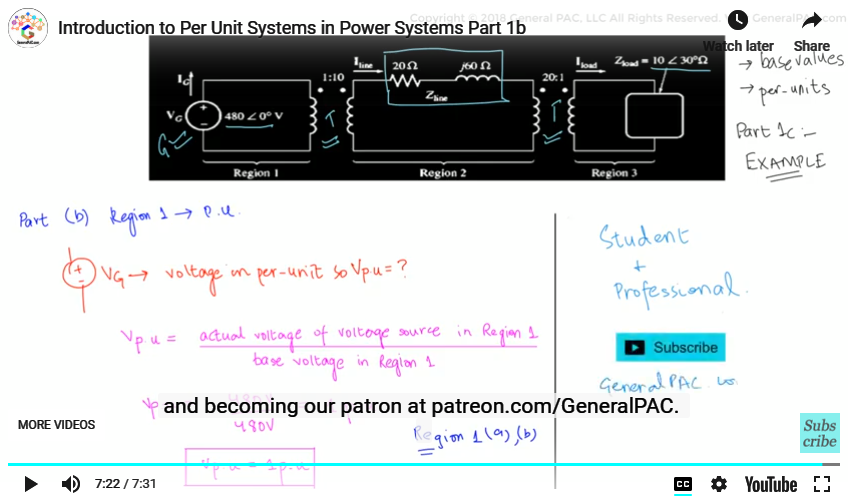GeneralPAC.com. Making Power Systems Intuitive, free and open to everyone, everywhere. Thank you.

#### Greetings from the GeneralPAC Team!

We make high-quality Power Systems Video Tutorials on complex topics that are free and open to everyone!  Thank you so much for supporting us through Patreon so we can continue doing good and valuable work.

What is Patreon and why do we use it?

Patreon is a fantastic portal that allows our fans and community to make monthly contribution (like Netflix subscription) so we can continue creating high-quality power systems video tutorials. In return, you get access to incredible perks like voting on future topics, getting your questions answered, access to VIP Q/A webinars with the creators of GeneralPAC, and much more! We THANK YOU for supporting us

Why do we need your support?

An incredible amount of time and effort is needed to develop high-quality video tutorials. Each video (Part 1 for example) takes approximately 10 hours to complete which includes learning the concept ourselves, brainstorming creative ways to teach and explain the concepts, writing the script, audio recording, video recording, and editing. It's no wonder why Hundreds-of-Thousands of people have watched, liked, subscribed, and left positive comments on Youtube channel. Your support truly makes all the difference.